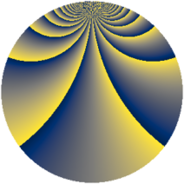# Properties

 Label 1600.2.cbLevel $1600$ Weight $2$ Character orbit 1600.cb Rep. character $\chi_{1600}(209,\cdot)$ Character field $\Q(\zeta_{20})$ Dimension $464$ Sturm bound $480$

# Related objects

## Defining parameters

 Level: $$N$$ $$=$$ $$1600 = 2^{6} \cdot 5^{2}$$ Weight: $$k$$ $$=$$ $$2$$ Character orbit: $$[\chi]$$ $$=$$ 1600.cb (of order $$20$$ and degree $$8$$) Character conductor: $$\operatorname{cond}(\chi)$$ $$=$$ $$400$$ Character field: $$\Q(\zeta_{20})$$ Sturm bound: $$480$$

## Dimensions

The following table gives the dimensions of various subspaces of $$M_{2}(1600, [\chi])$$.

Total New Old
Modular forms 1984 496 1488
Cusp forms 1856 464 1392
Eisenstein series 128 32 96

## Trace form

 $$464q + 10q^{3} - 8q^{5} + O(q^{10})$$ $$464q + 10q^{3} - 8q^{5} + 6q^{11} - 10q^{13} + 16q^{15} - 20q^{17} + 6q^{19} - 24q^{21} + 10q^{27} - 6q^{29} + 36q^{31} - 20q^{33} + 36q^{35} - 10q^{37} - 24q^{45} + 20q^{47} + 336q^{49} + 28q^{51} - 10q^{53} + 6q^{59} - 6q^{61} + 160q^{63} - 48q^{65} + 70q^{67} + 6q^{69} + 46q^{75} - 80q^{77} + 52q^{79} + 72q^{81} + 10q^{83} - 18q^{85} + 48q^{91} + 40q^{95} - 20q^{97} + 64q^{99} + O(q^{100})$$

## Decomposition of $$S_{2}^{\mathrm{new}}(1600, [\chi])$$ into newform subspaces

The newforms in this space have not yet been added to the LMFDB.

## Decomposition of $$S_{2}^{\mathrm{old}}(1600, [\chi])$$ into lower level spaces

$$S_{2}^{\mathrm{old}}(1600, [\chi]) \cong$$ $$S_{2}^{\mathrm{new}}(400, [\chi])$$$$^{\oplus 3}$$